# Mathaid precalculus

Highly interactive tutorials and self-test system for individual e-learning, home schooling, college and high school computer learning centers, and distance learning. The product covers the standard topics of a one-year high school course preparing for Calculus, with the emphasis on building problem-solving skills. Tutorials include the reviews of basic concepts, interactive examples, and standard problems with randomly generated parameters. The self-test system allows selecting topics and length for a test, saving test results, and getting the test review. Topics covered: rectangular coordinate system, functions and graphs, linear and absolute value functions, slope of a line, equations of straight lines, systems of linear equations and inequalities, determinants and Cramer's rule, polynomial and rational functions, complex numbers, quadratic functions, conic sections, exponential and logarithmic functions, angles and their measure, trigonometric functions, trigonometric identities,

## Related software (5)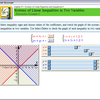## MathAid Algebra II

Tutorial contains basic concepts, interactive examples, and problems with randomly generated parameters. A customer is allowed to select chapters for a test, get the test review, ...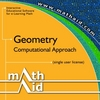## MathAid Geometry

Tutorial contains basic concepts, interactive examples, and problems with randomly generated parameters. A customer is allowed to select chapters for a test, get the test review, ...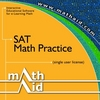## MathAid SAT. Math Practice

Tutorial contains basic concepts, interactive examples, and problems with randomly generated parameters. A customer is allowed to select chapters for a test, get the test review, ...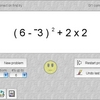## Smooth Operators

Smooth Operators is a complete solution for learning, practicing, and testing the order of operations. An interactive lesson teaches concepts. Practice by clicking operands, or tes ...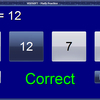## Math Practice

Math Practice is an easy to use software addressed to parents who wish to help kids make their first steps into the world of math. Choose between addition, subtraction, multiplicat ...